# 看图学 NumPy：掌握 n 维数组基础知识点，看这一篇就够了NumPy 是 Python 的最重要的扩展程序库之一，也是入门机器学习编程的必备工具。然而对初学者来说，NumPy 的大量运算方法非常难记。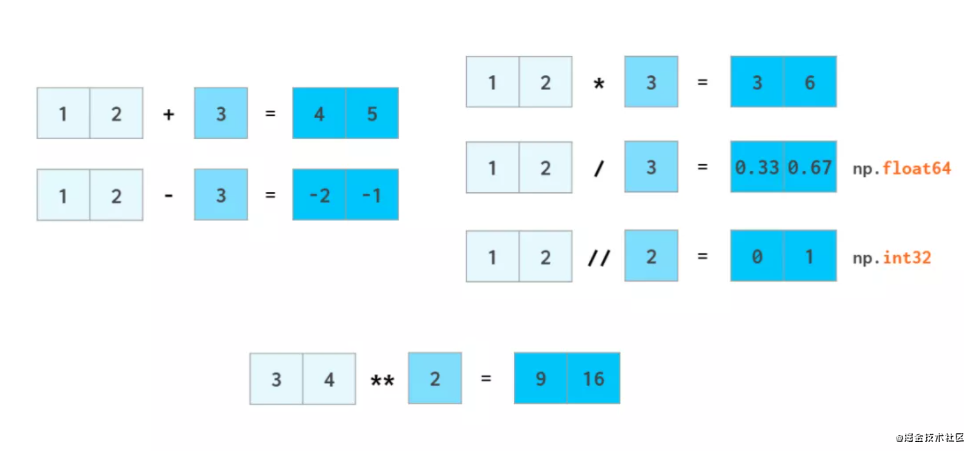## Numpy 数组与 Python 列表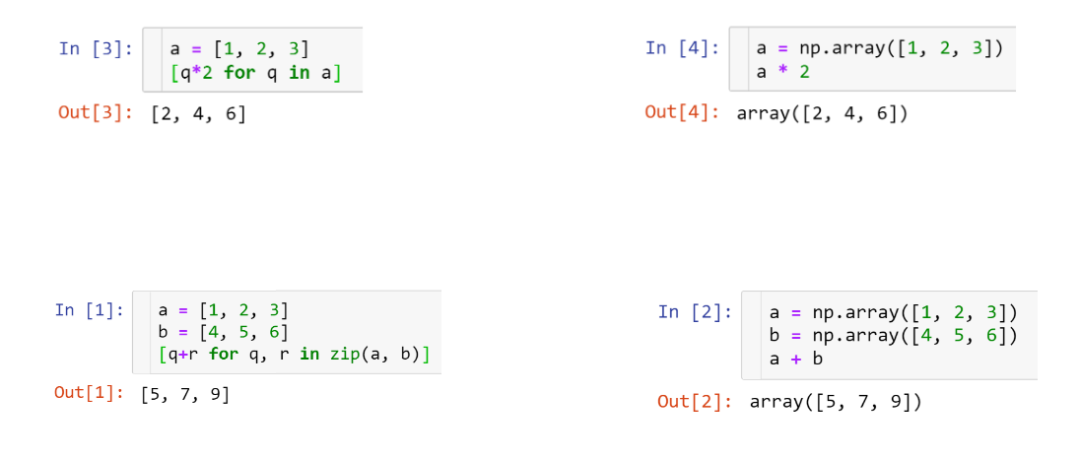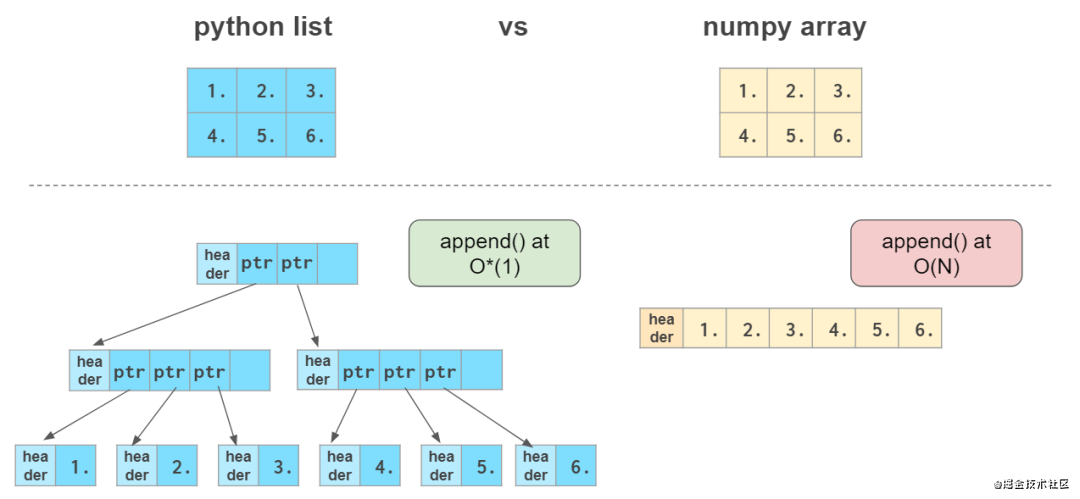## 向量运算

#### 向量初始化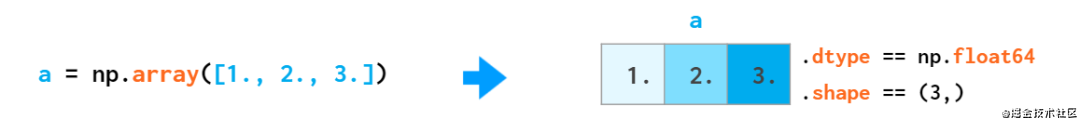NumPy 数组无法像 Python 列表那样加长，因为在数组末尾没有保留空间。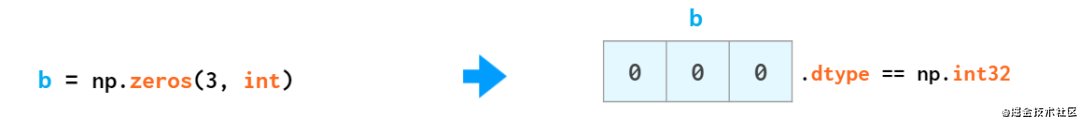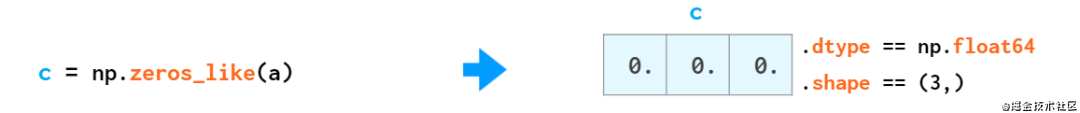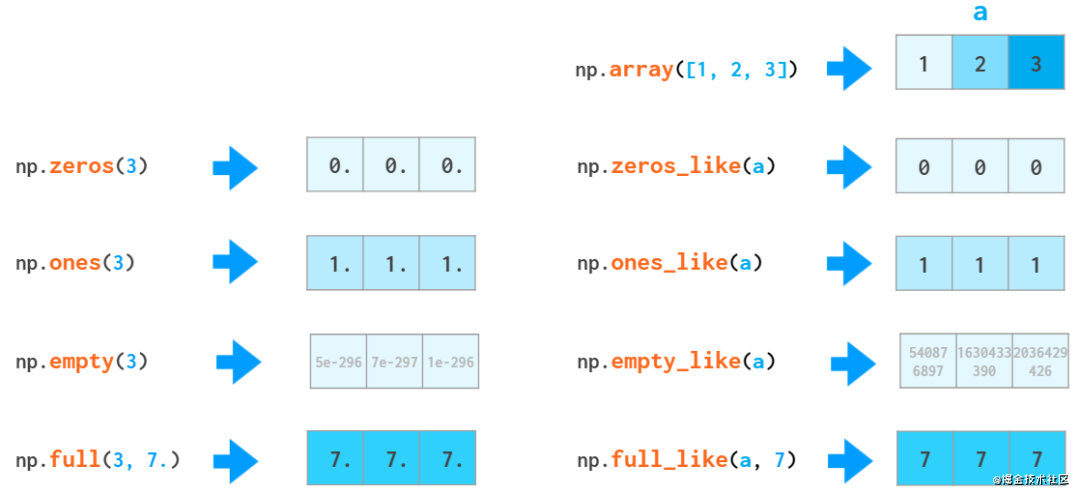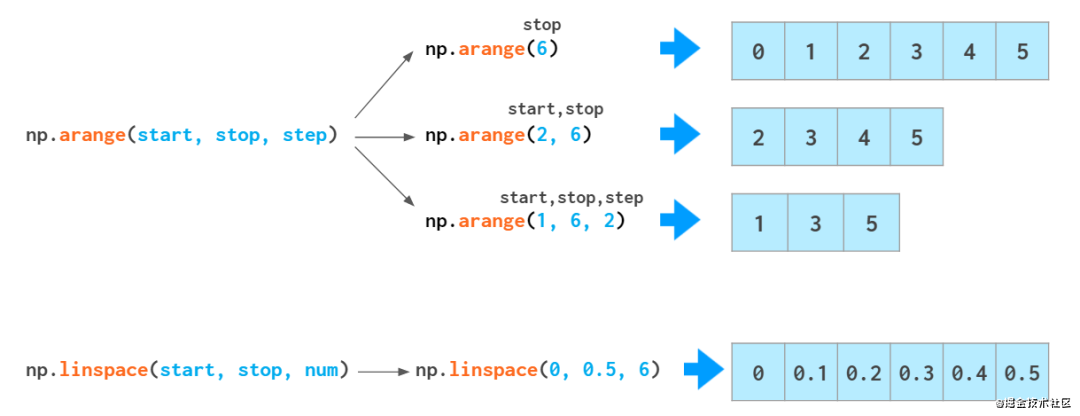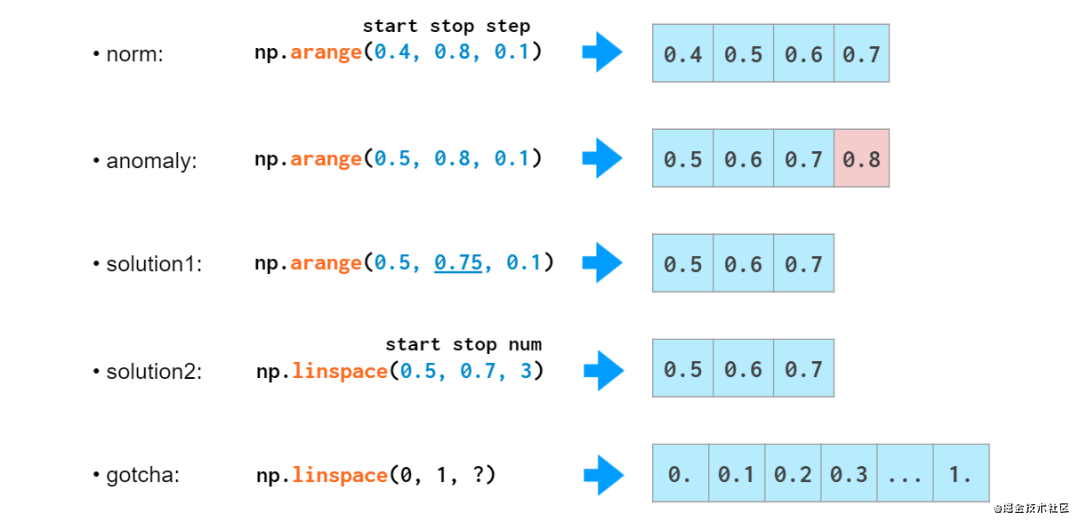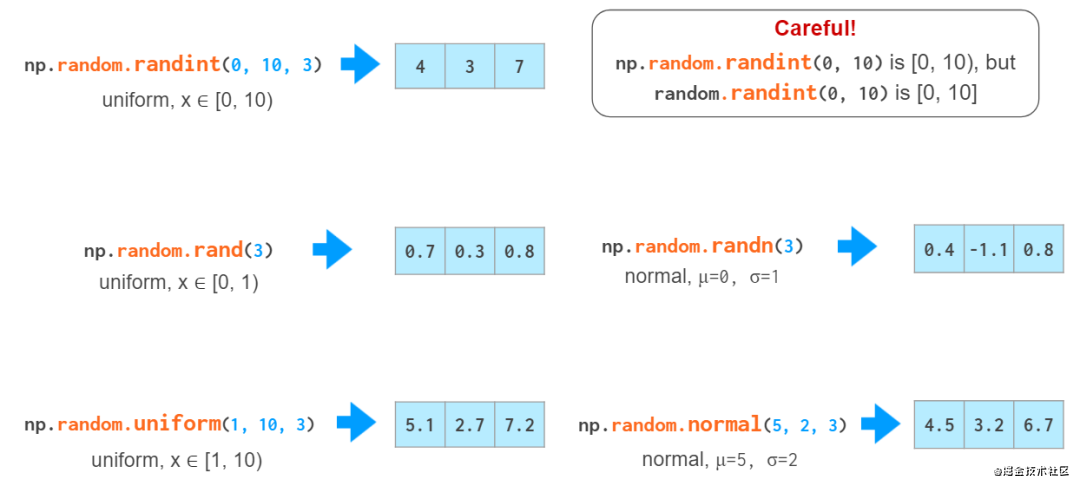#### 向量索引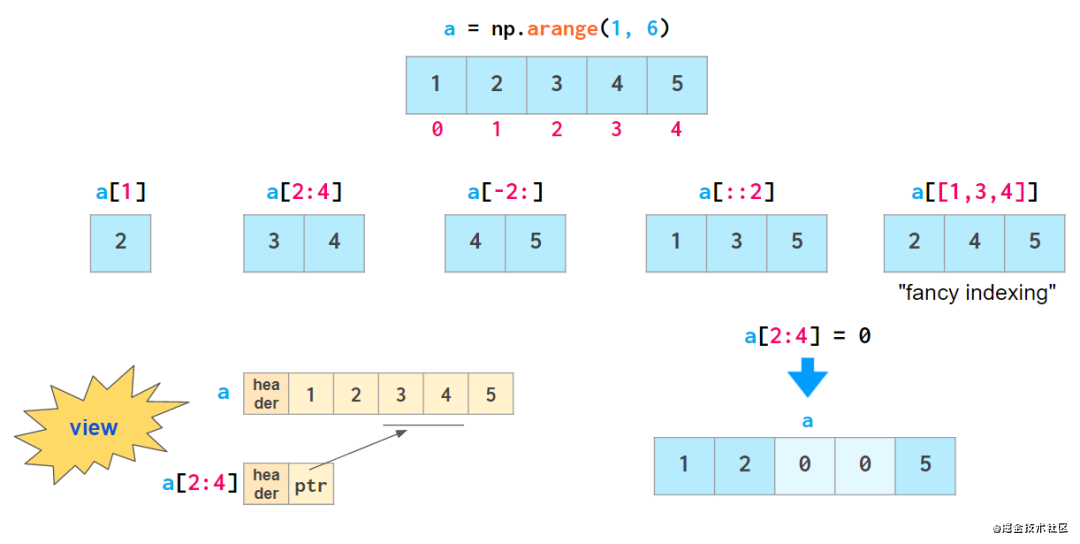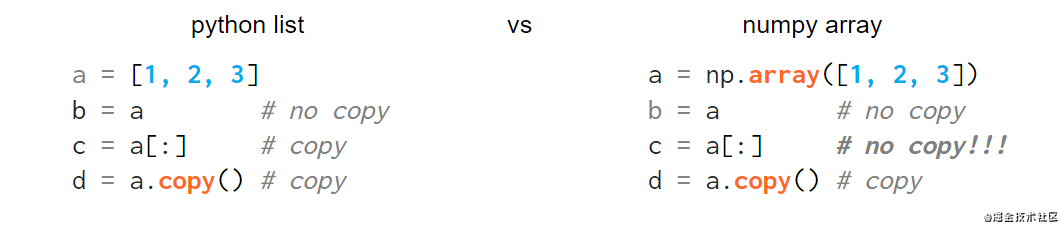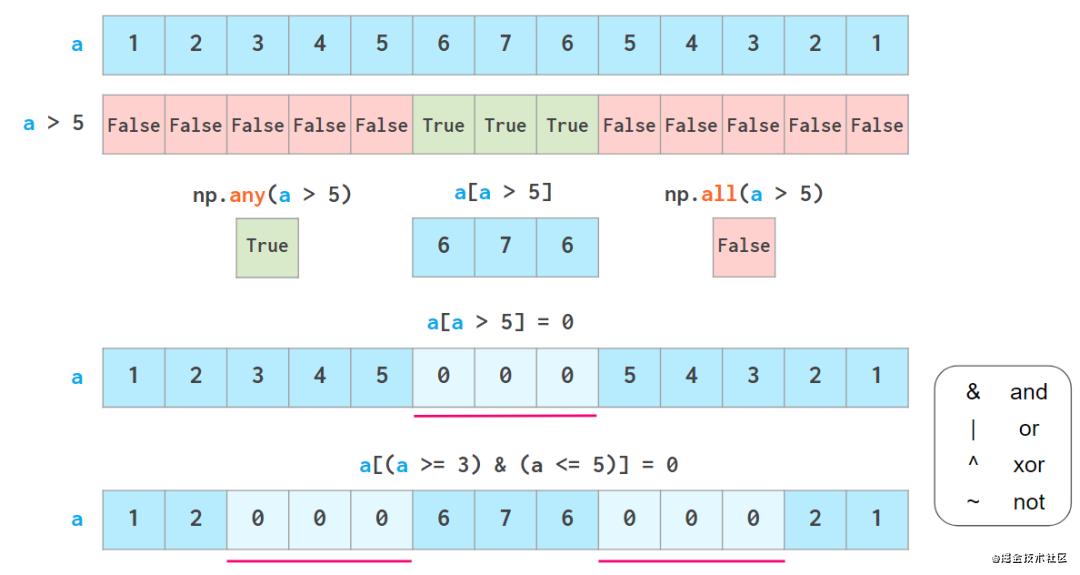#### 向量运算

NumPy 允许像普通数字一样操作整个数组（加减乘除、整除、幂）：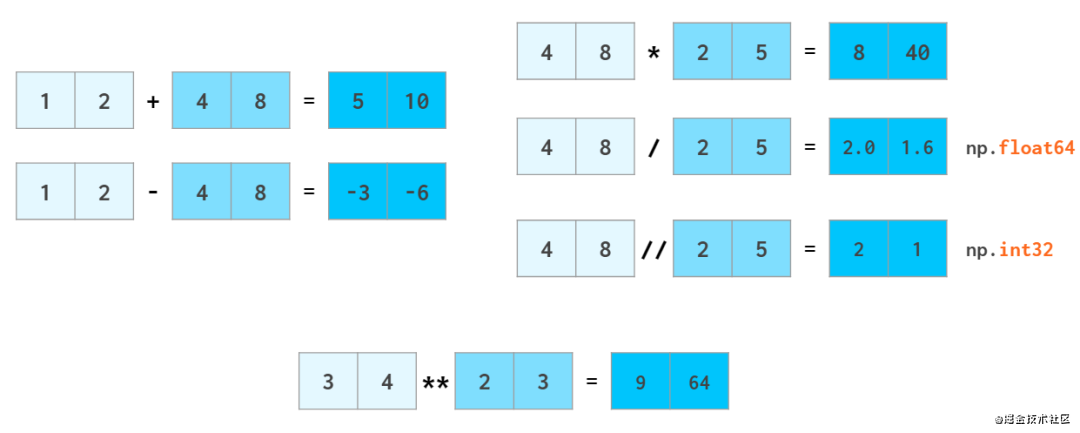△ 和 Python 中一样，a//b 表示 div b（整除），x**n 表示 xⁿ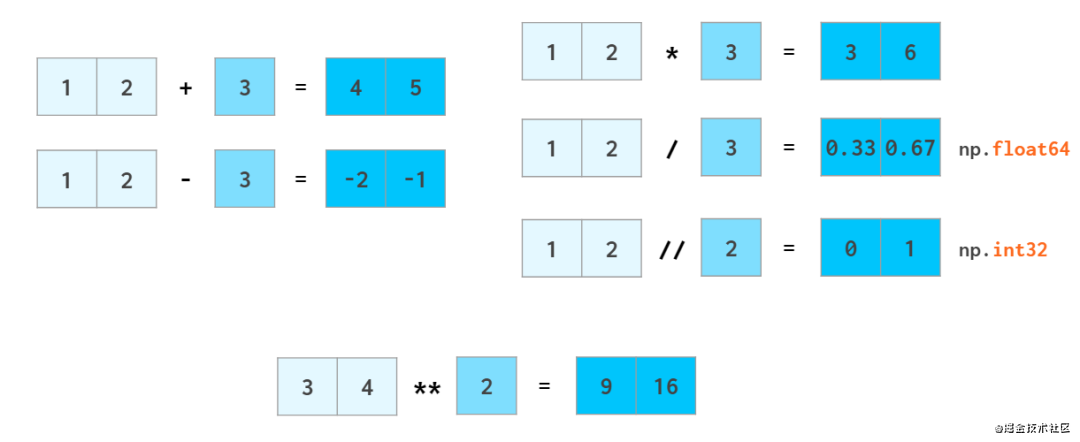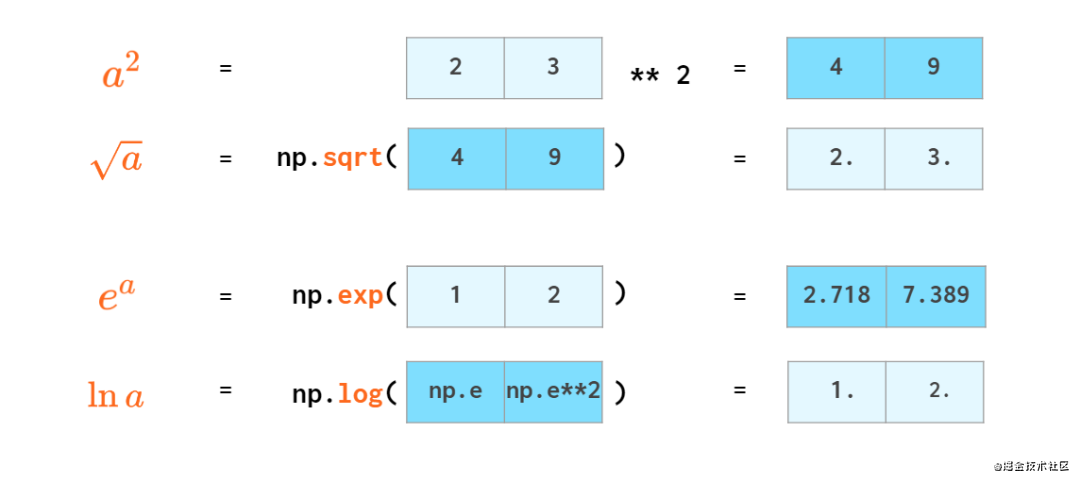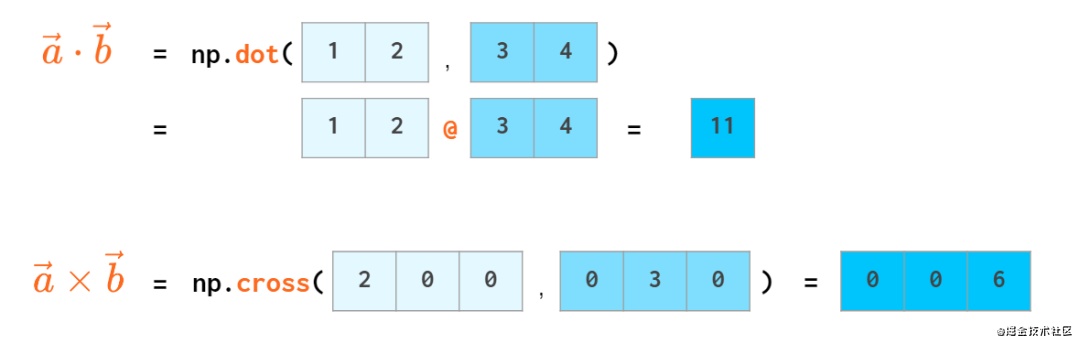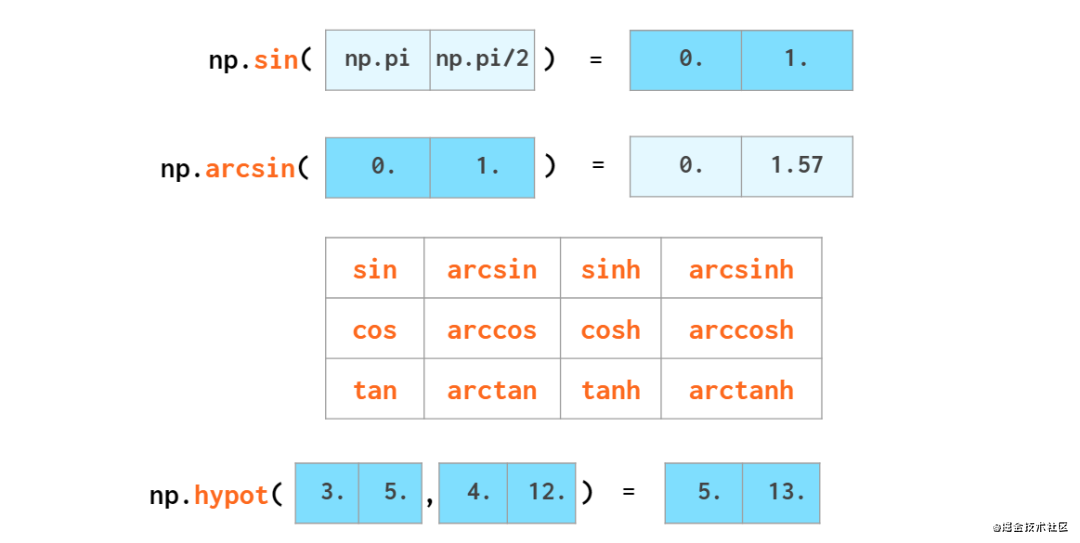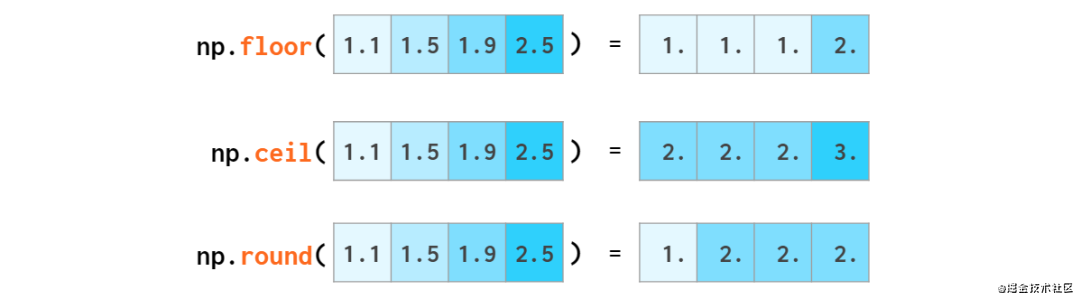△ floor 取下界；ceil 取上界；round 为四舍六入五取偶

NumPy 还可以执行以下基本的统计运算（最大最小值、平均值、方差、标准差）：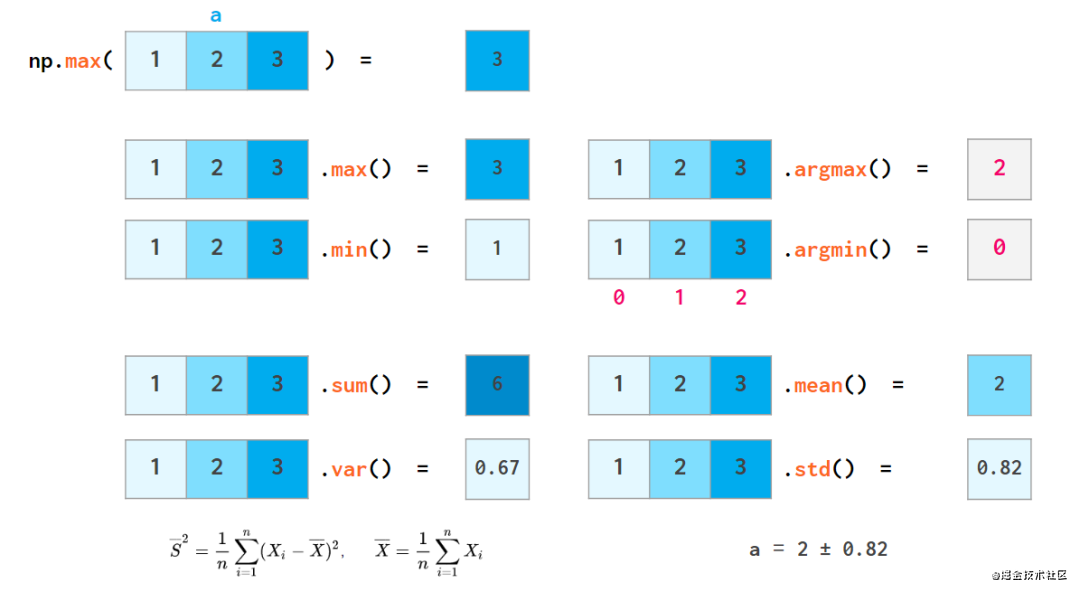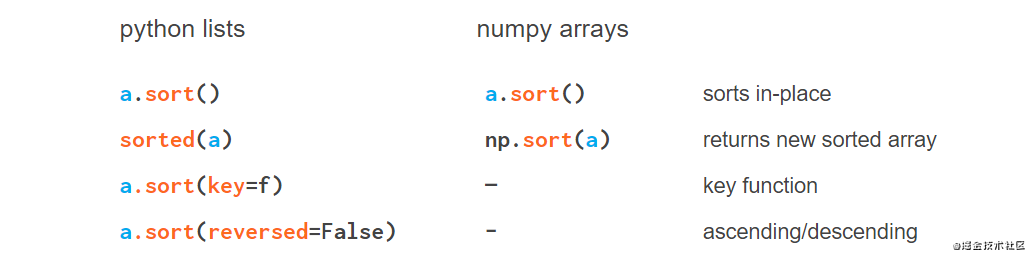#### 搜索向量中的元素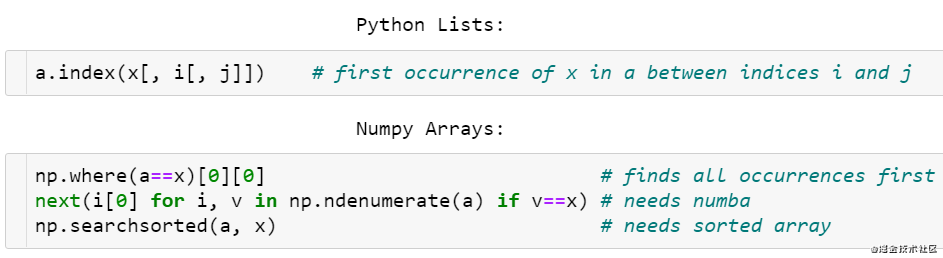• 查找元素的一种方法是 np.where(a==x)0，它既不优雅也不快速，因为要查找的项需要从开头遍历数组的所有元素。

• 更快的方式是通过 Numba 中的 next((i for i, v in np.ndenumerate(a) if v==x), -1)来加速。

• 一旦对数组进行排序，情况就会变得更好：v = np.searchsorted(a, x); return v if a[v]==x else -1 的复杂度为 O(log N)，确实非常快，但是首先需要 O(N log N)的排序时间。

#### 比较浮点数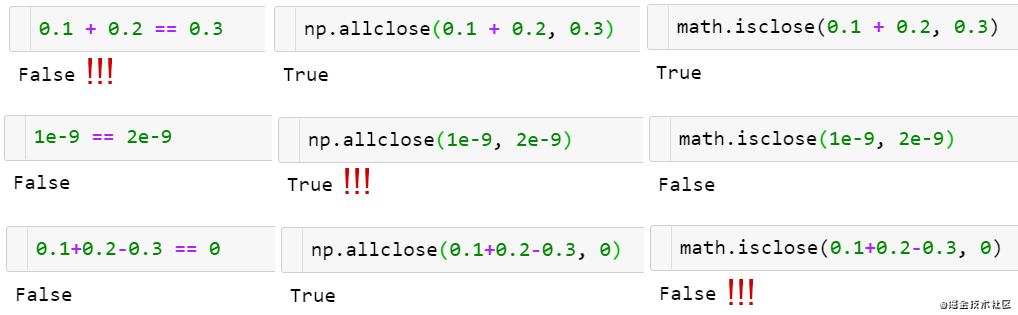• np.allclose 假设所有的比较数字的等级是 1 个单位。例如在上图中，它就认为 1e-9 和 2e-9 相同，如果要进行更细致的比较，需要通过 atol 指定比较等级 1：np.allclose(1e-9, 2e-9, atol=1e-17) == False。

• math.isclose 进行比较没有假设前提，而是基于用户给出的一个合理 abs_tol 值：math.isclose(0.1+0.2–0.3, abs_tol=1e-8) == True。

## 矩阵运算

NumPy 中曾经有一个专用的类 matrix，但现在已弃用，因此下面将交替使用矩阵和 2D 数组两个词。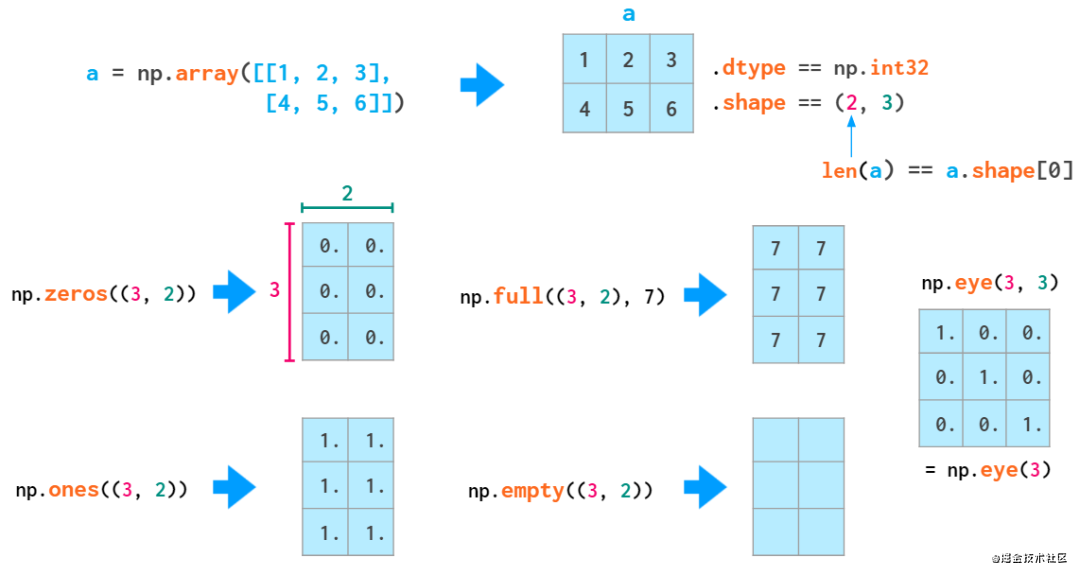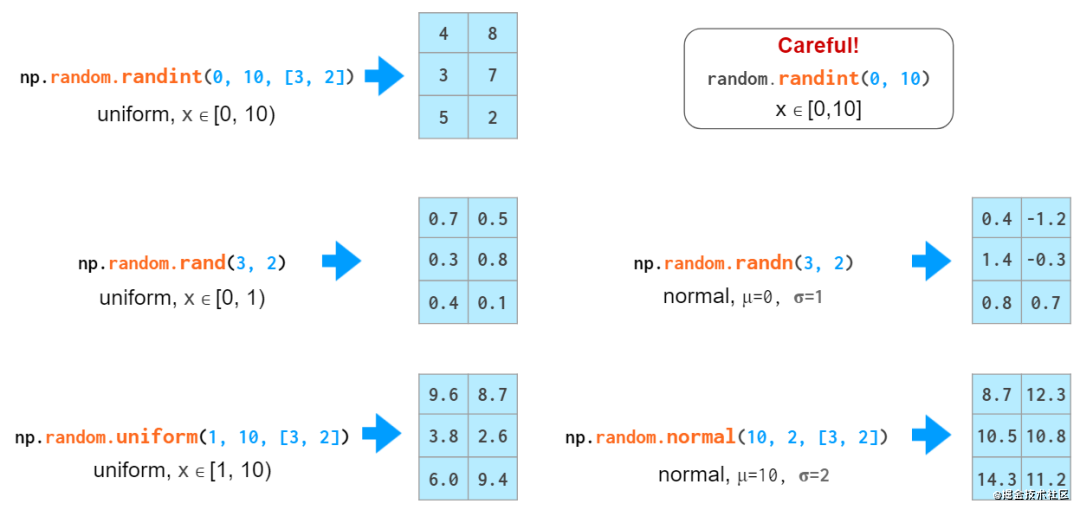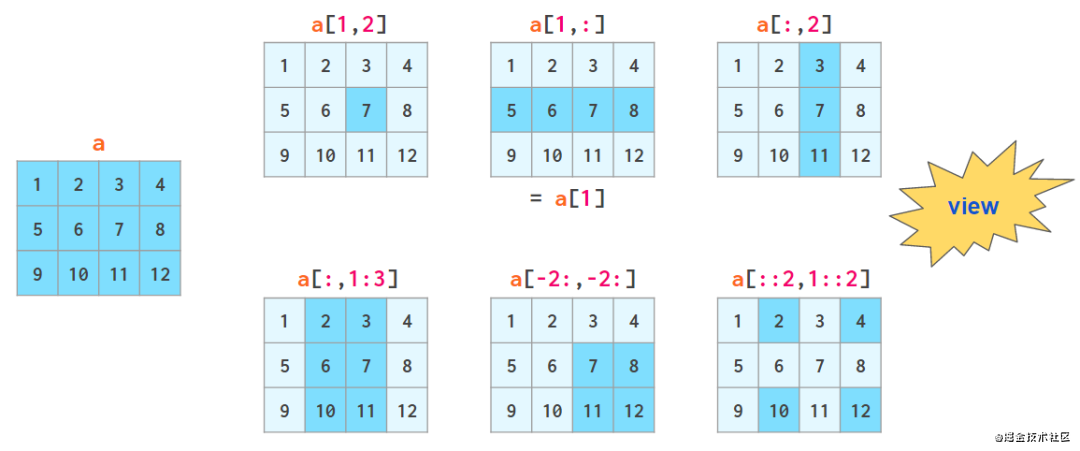#### axis 参数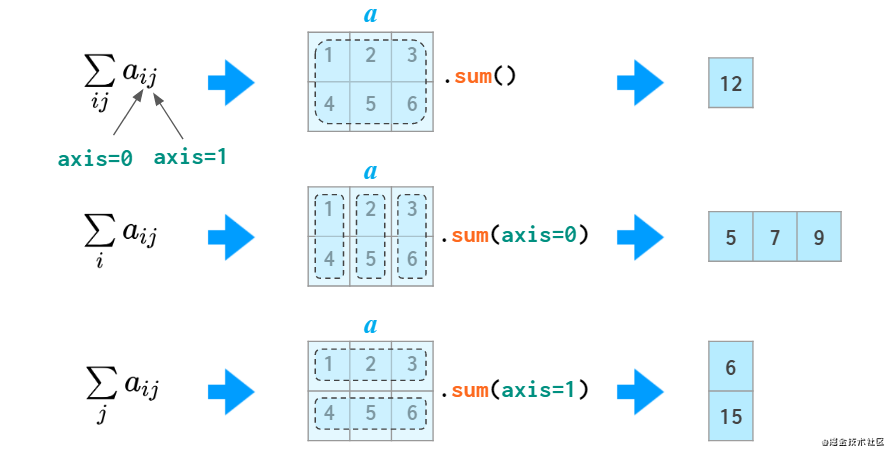#### 矩阵运算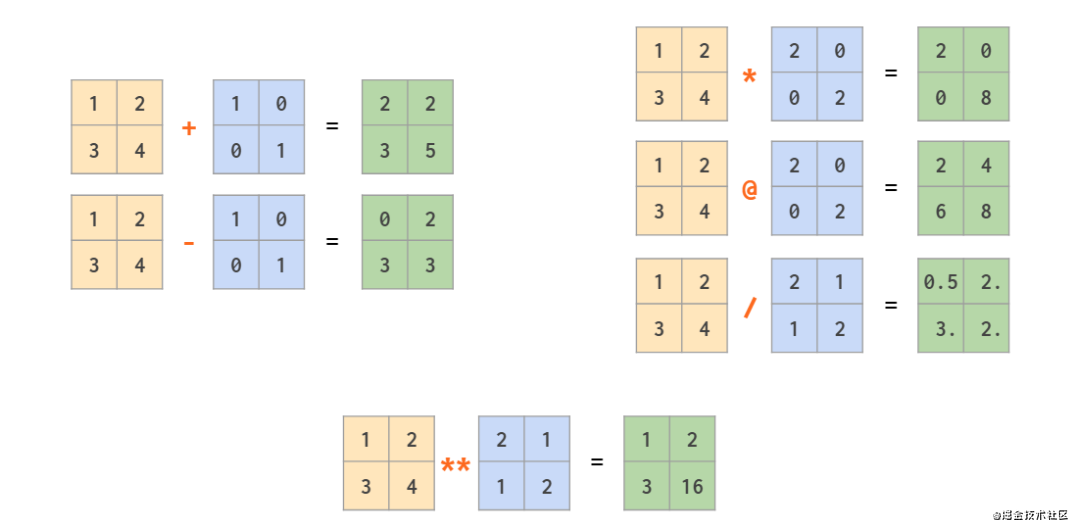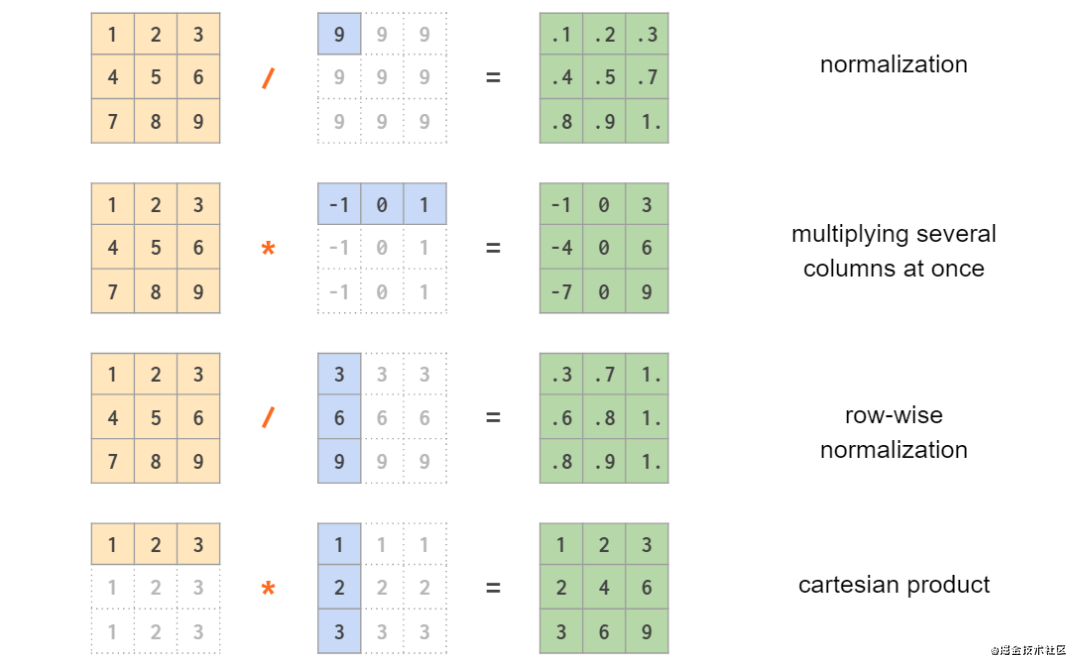#### 行向量与列向量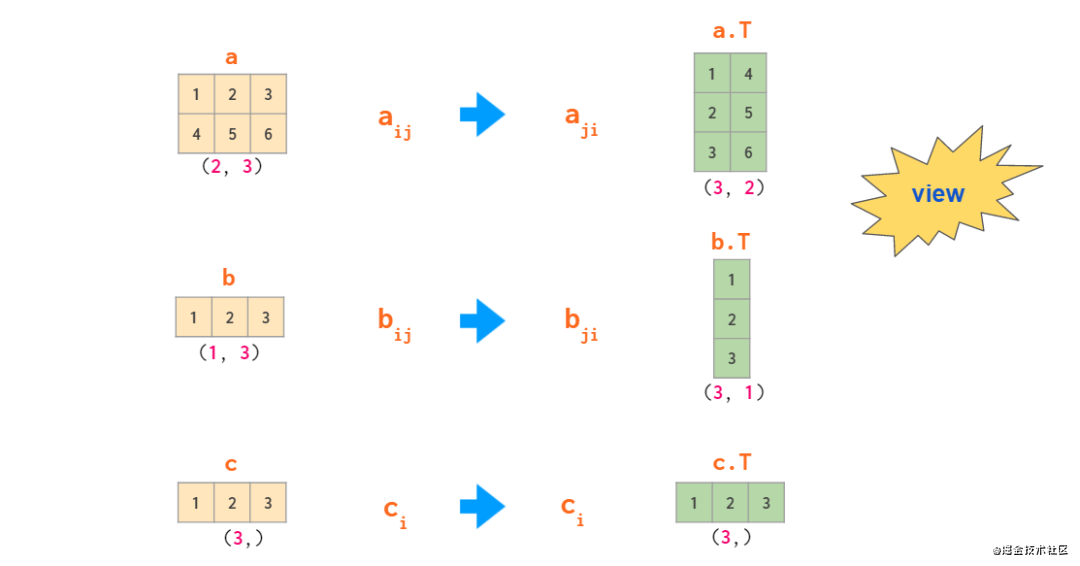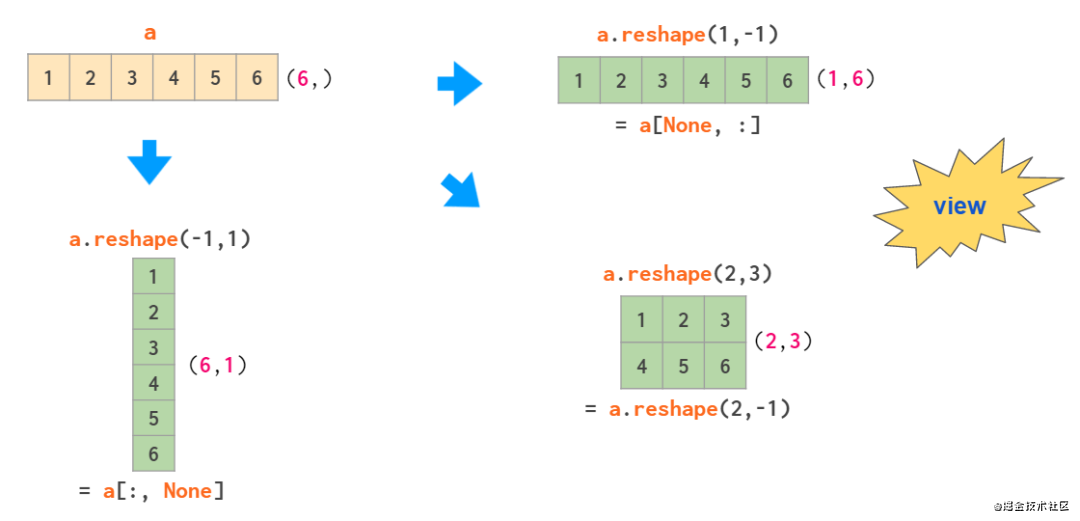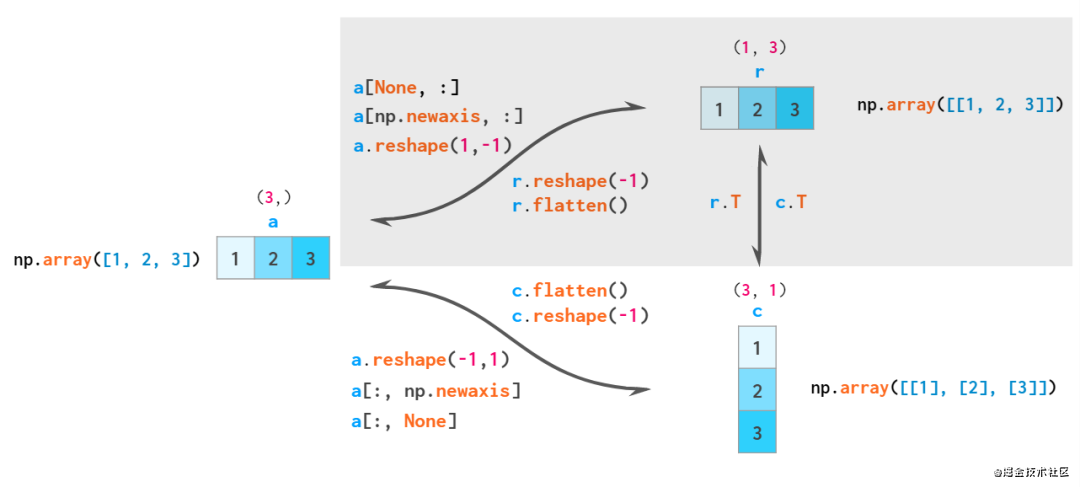#### 矩阵操作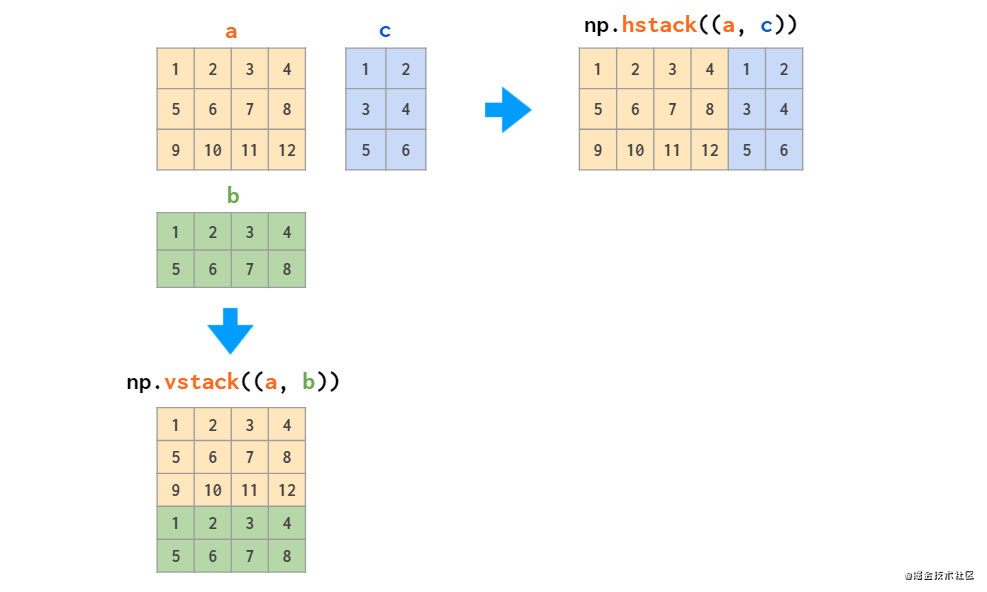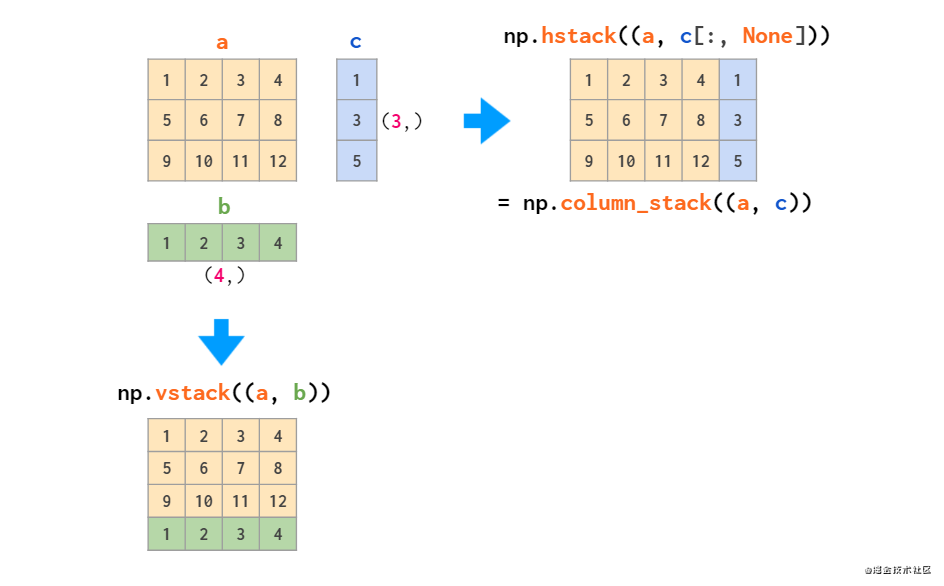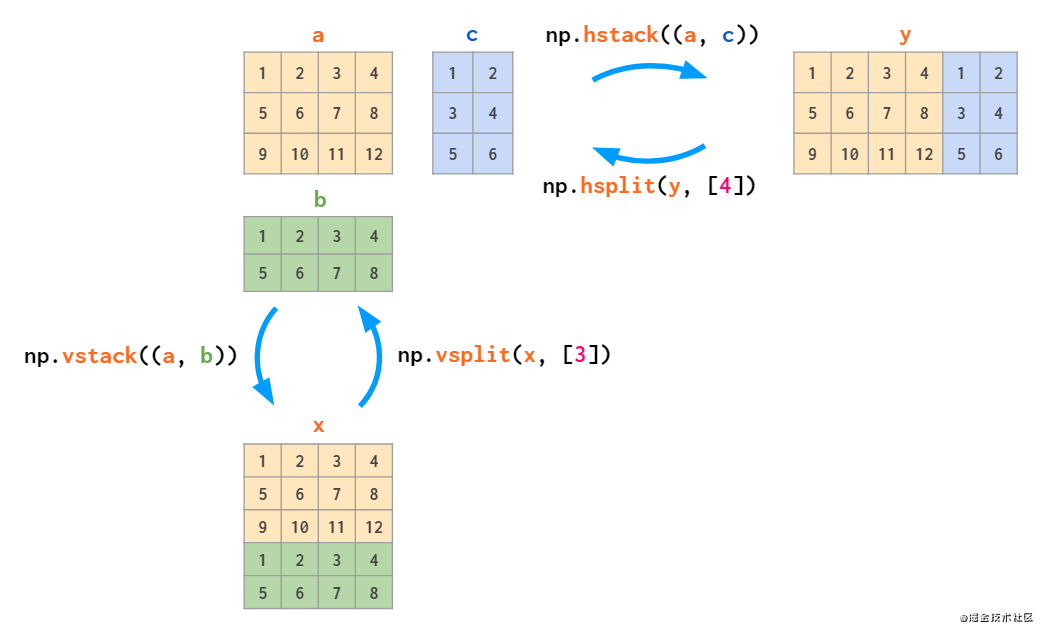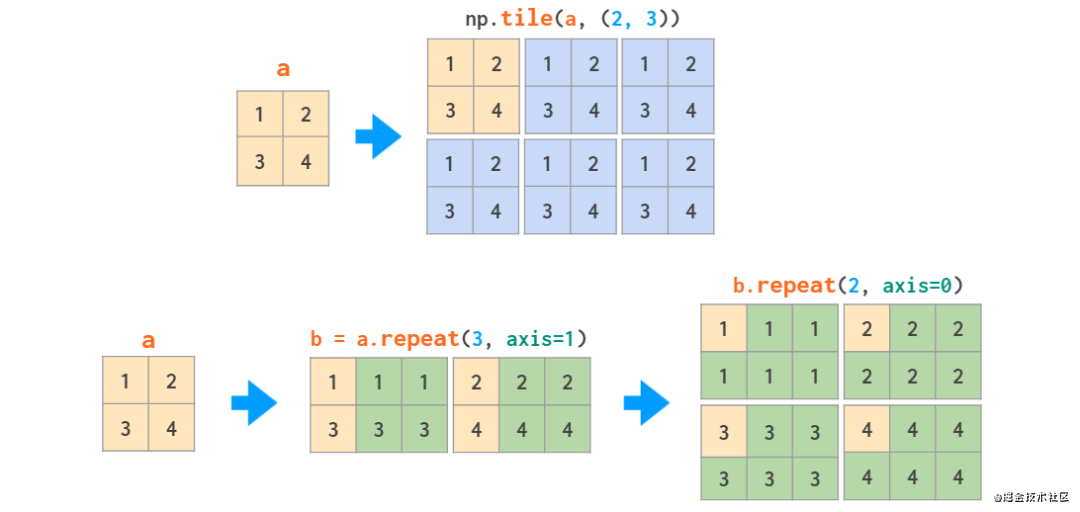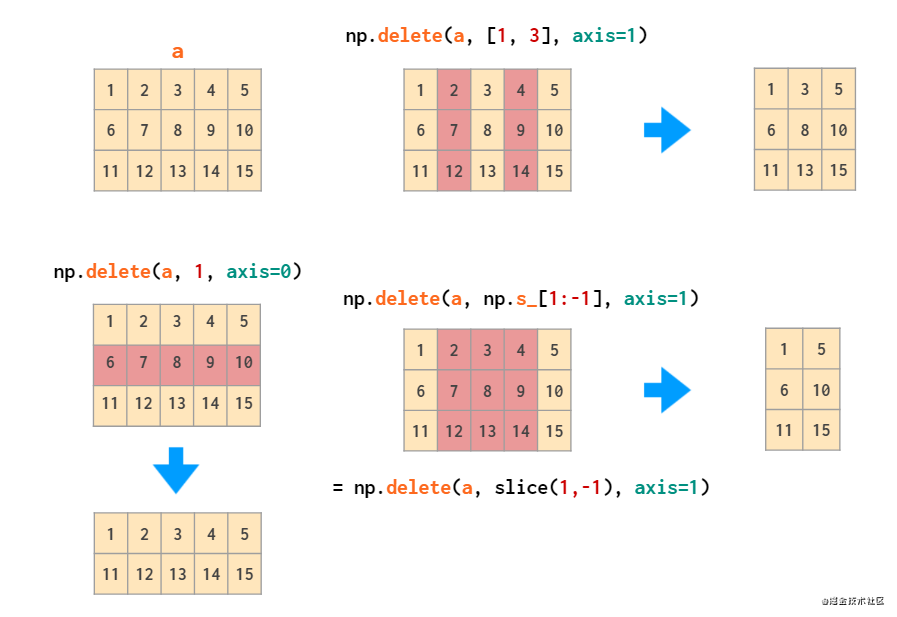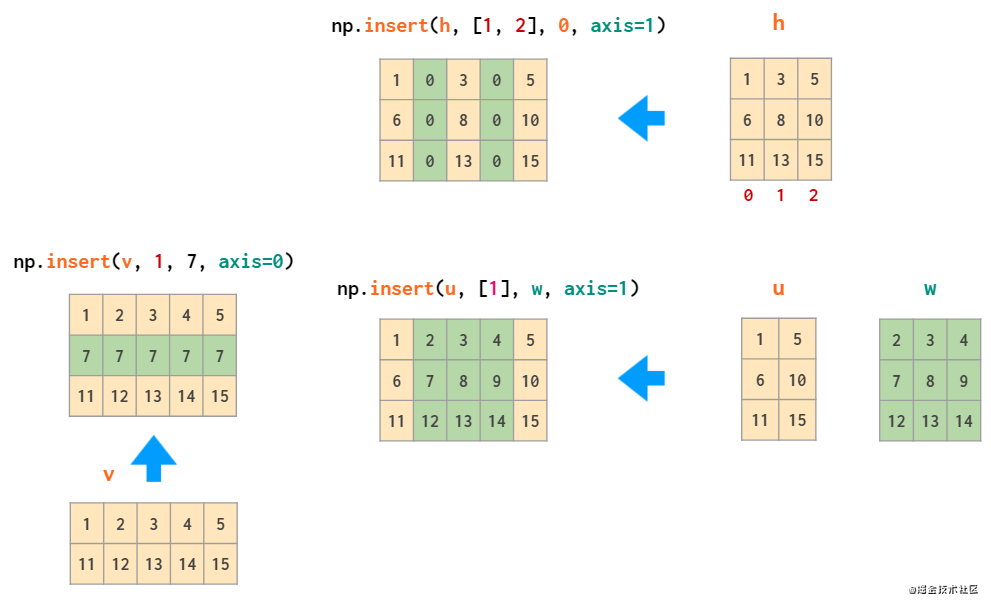append 就像 hstack 一样，该函数无法自动转置一维数组，因此再次需要对向量进行转置或添加长度，或者使用 column_stack 代替：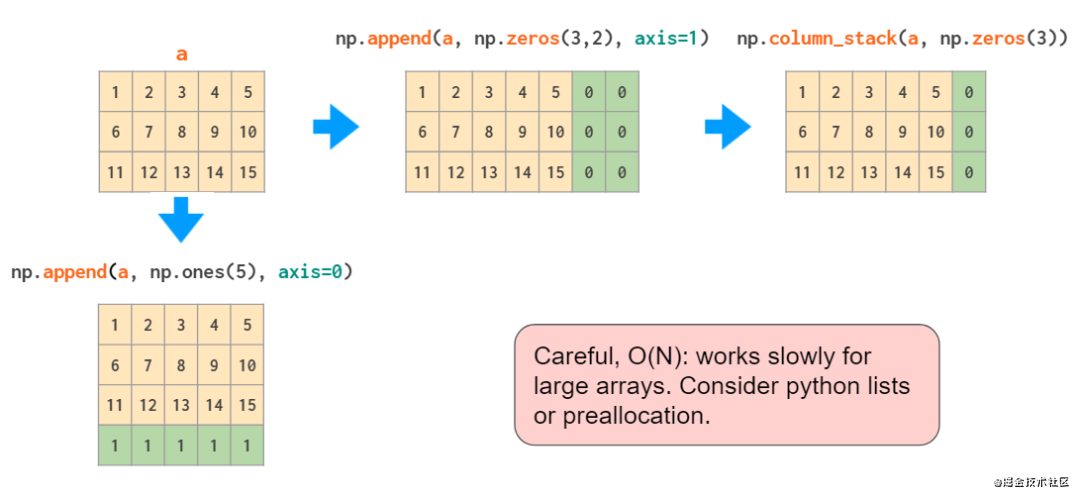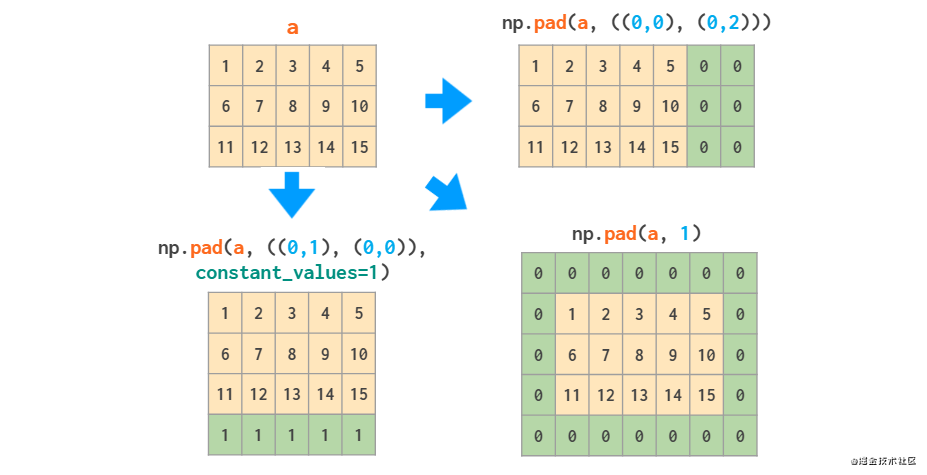#### Meshgrid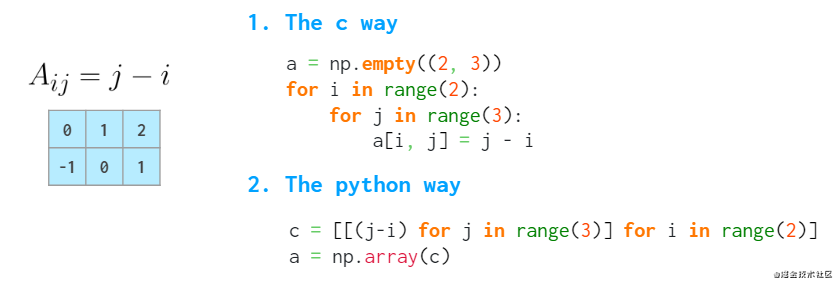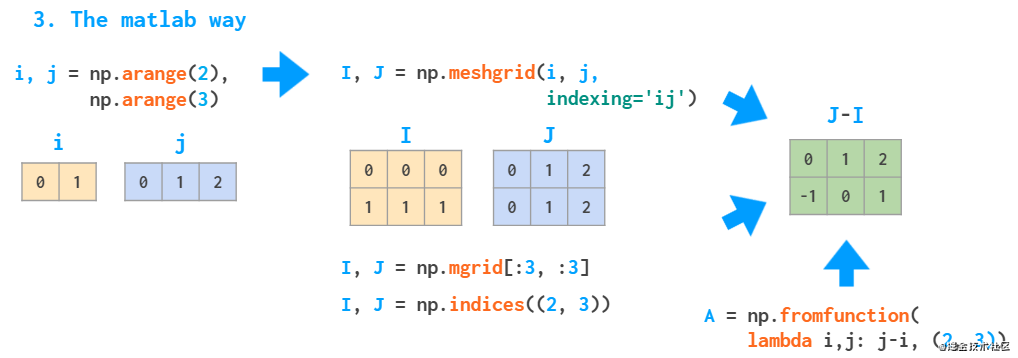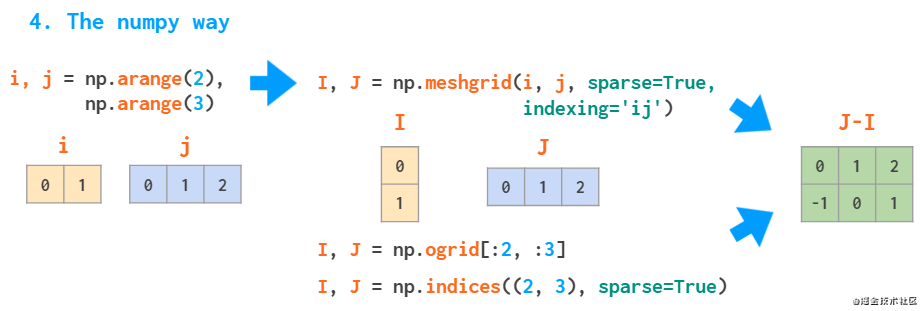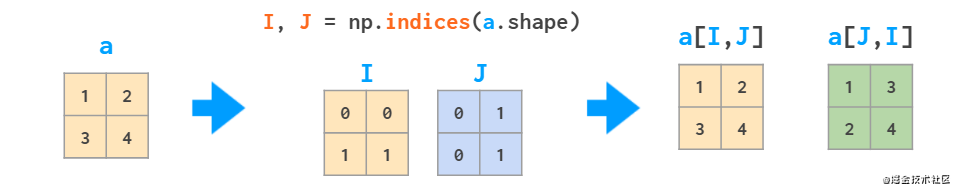#### 矩阵统计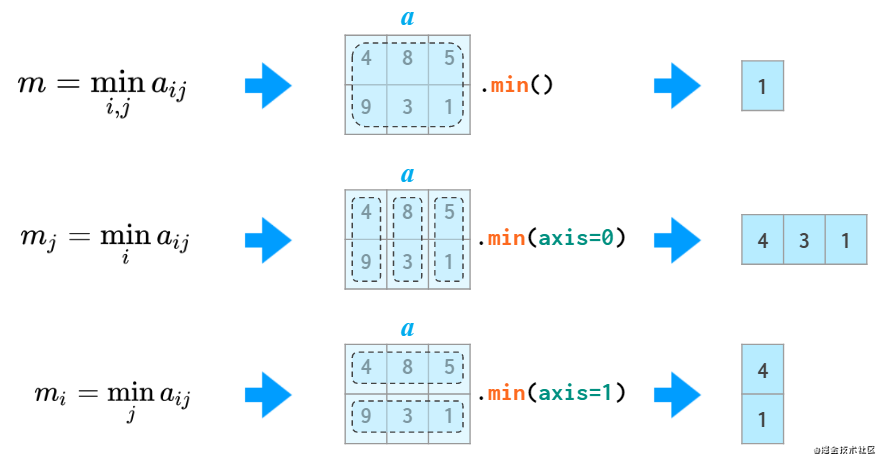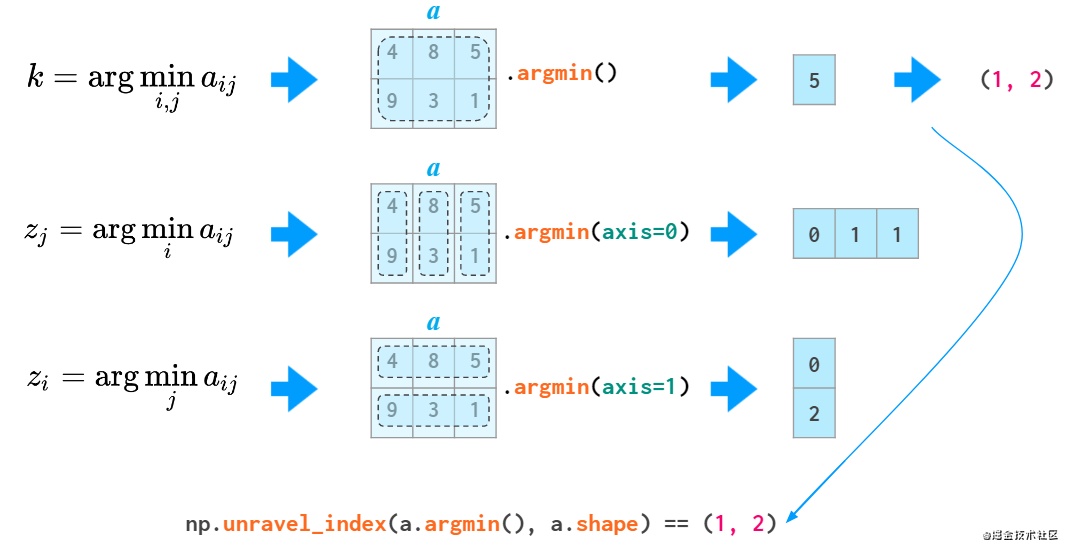all 和 any 两个函数也能使用 axis 参数：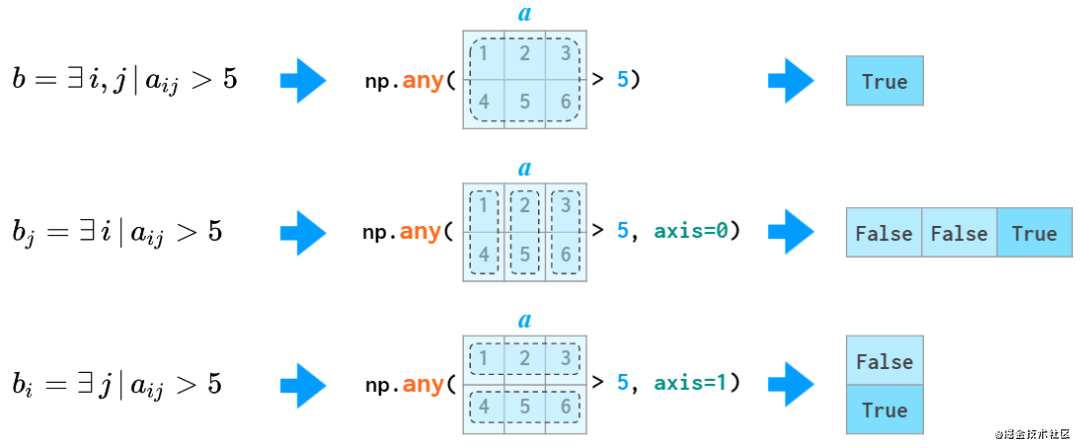#### 矩阵排序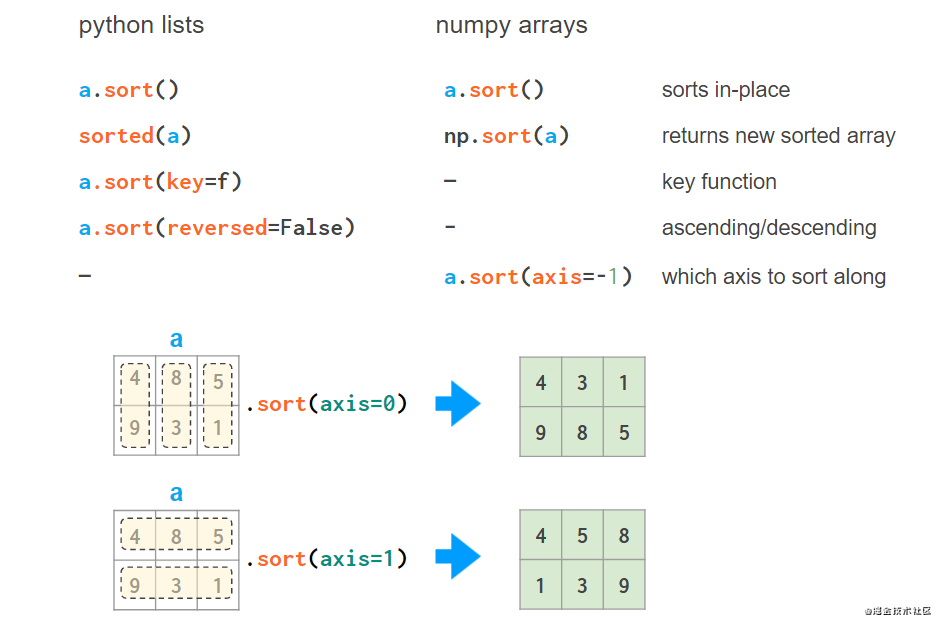axis 绝不是 Python 列表 key 参数的替代。不过 NumPy 具有多个函数，允许按列进行排序：

1、按第一列对数组排序：a[a[:,0].argsort()]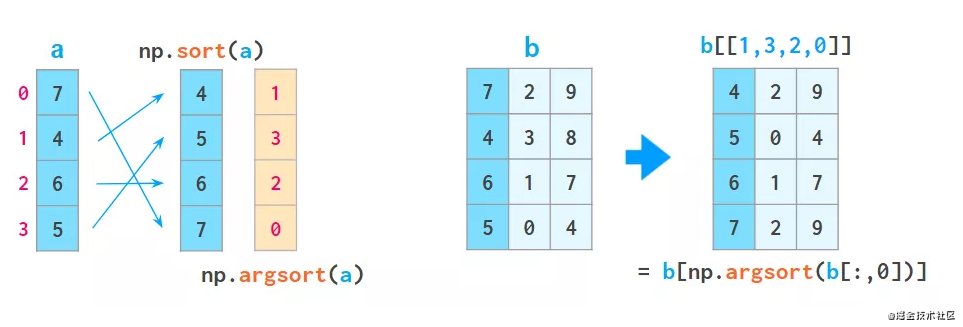argsort 排序后，此处返回原始数组的索引数组。

a = a[a[:,2].argsort()]a = a[a[:,1].argsort(kind=’stable’)]a = a[a[:,0].argsort(kind=’stable’)]

2、有一个辅助函数 lexsort，该函数按上述方式对所有可用列进行排序，但始终按行执行，例如：

• a[np.lexsort(np.flipud(a[2,5].T))]：先通过第 2 列排序，再通过第 5 列排序；

• a[np.lexsort(np.flipud(a.T))]：按从左到右所有列依次进行排序。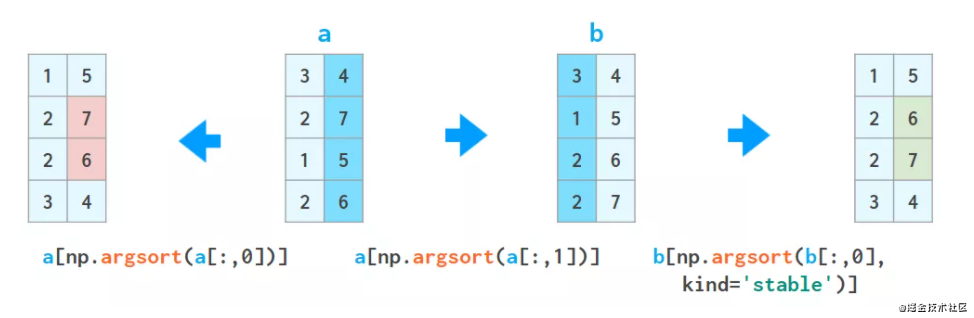3、还有一个参数 order，但是如果从普通（非结构化）数组开始，则既不快速也不容易使用。

4、因为这个特殊的操作方式更具可读性和它可能是一个更好的选择，这样做的 pandas 不易出错：

• pd.DataFrame(a).sort_values(by=[2,5]).to_numpy()：通过第 2 列再通过第 5 列进行排序。

• pd.DataFrame(a).sort_values().to_numpy()：通过从左向右所有列进行排序

## 高维数组运算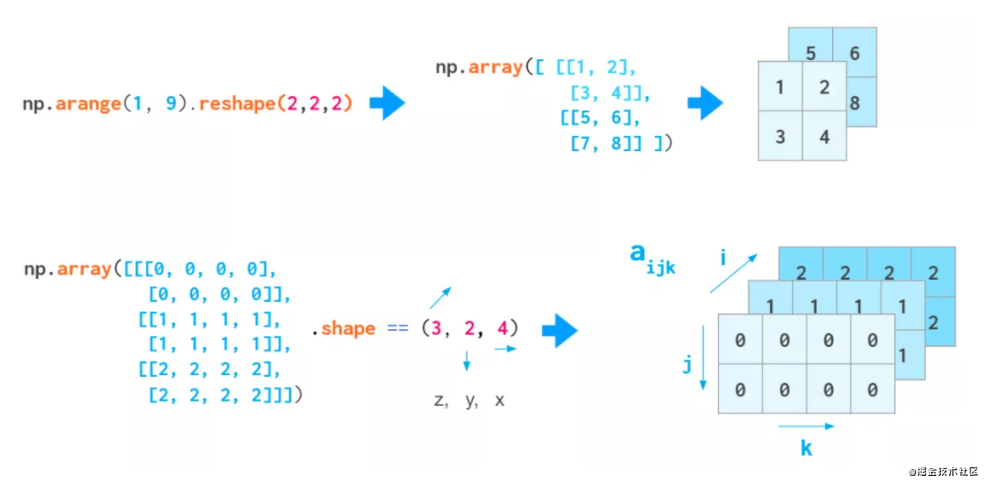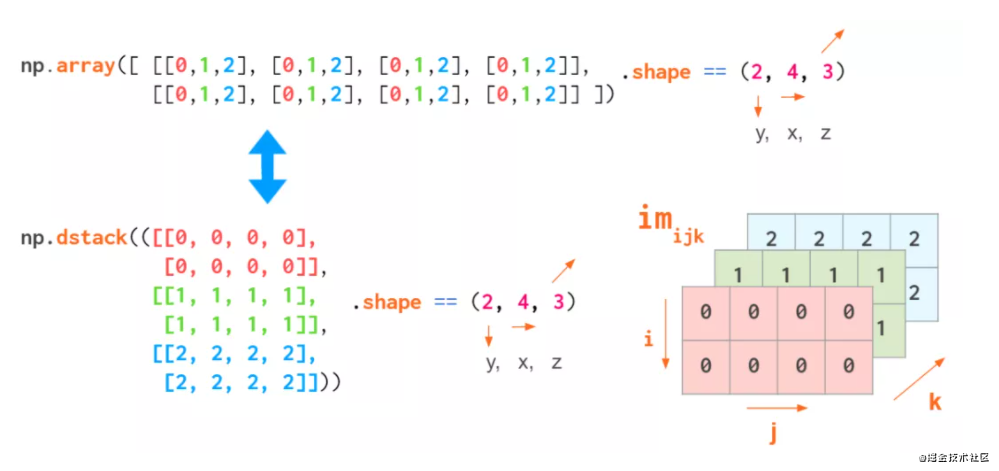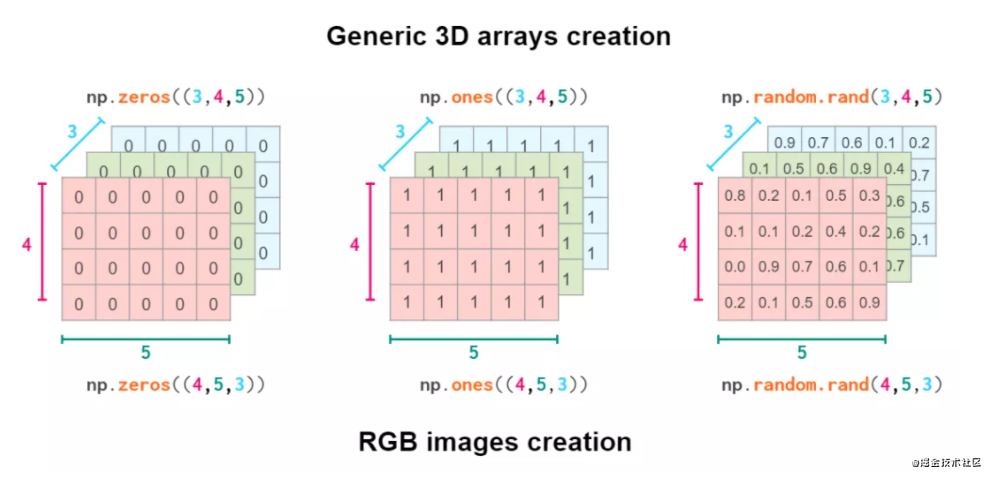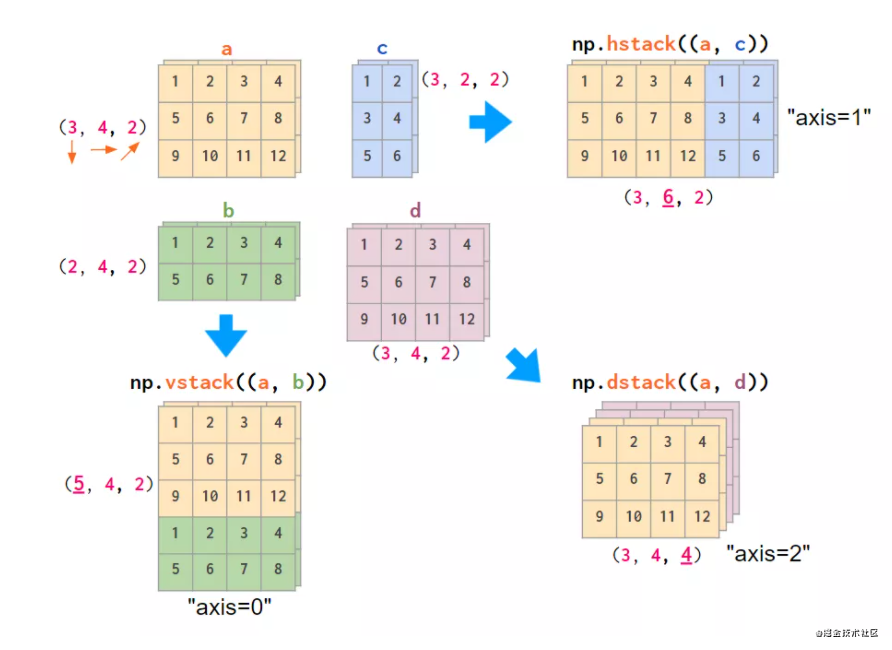△RGB 图像数组（为简便起见，上图仅 2 种颜色）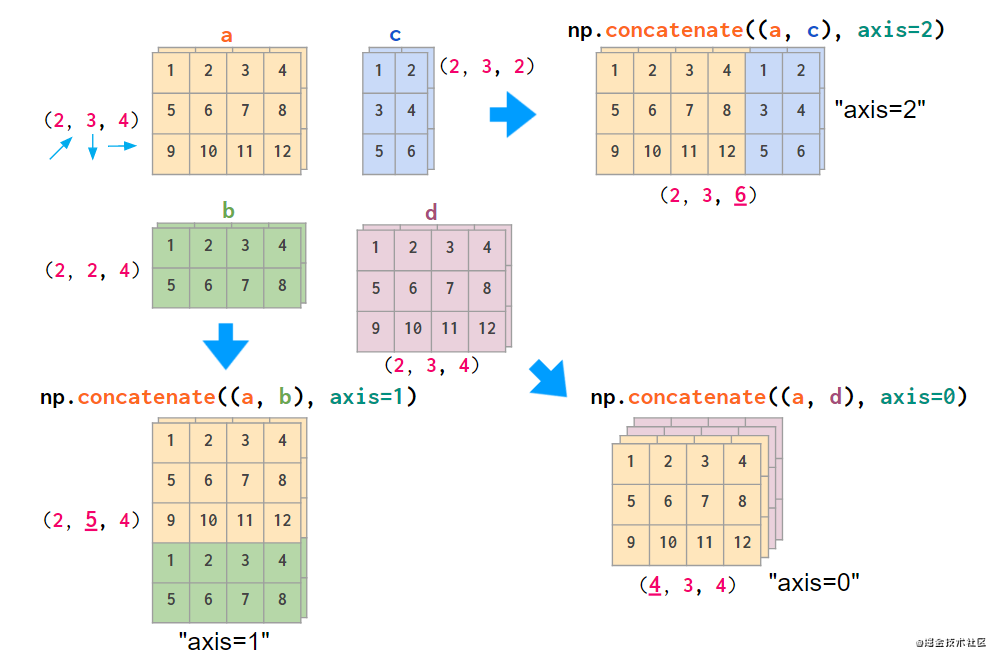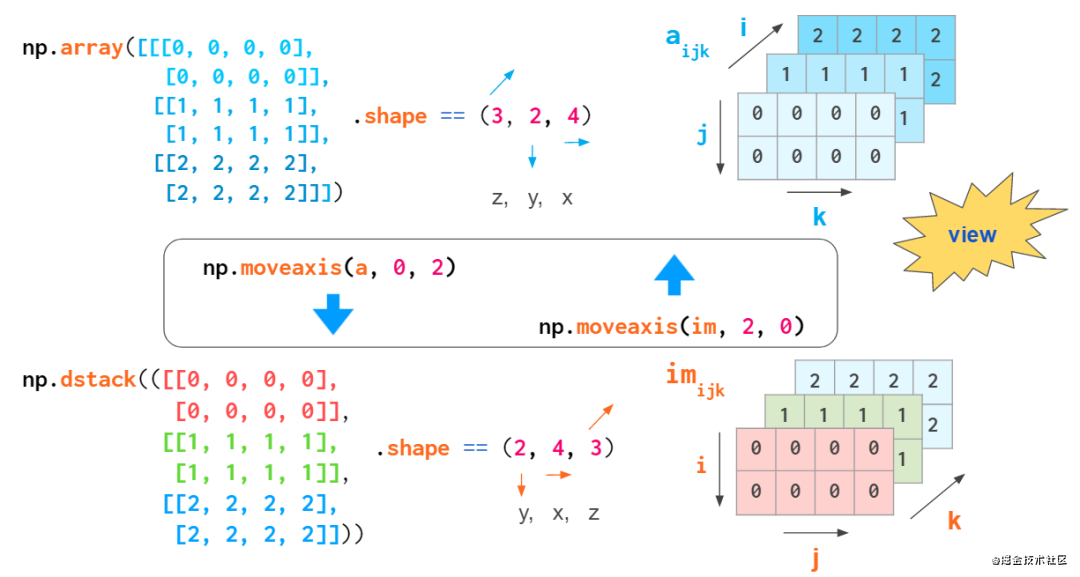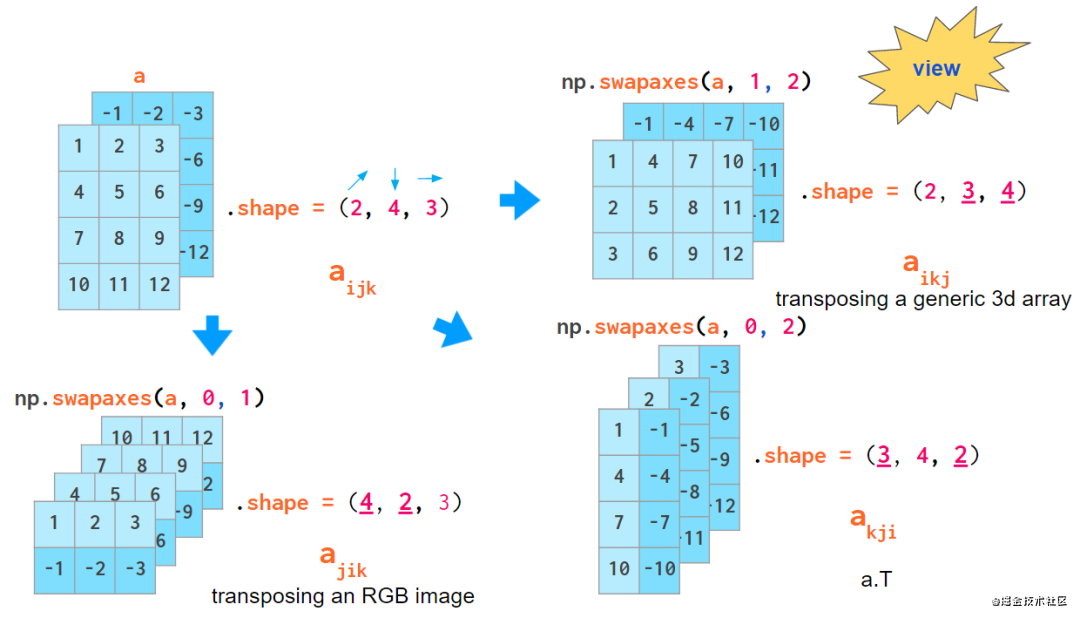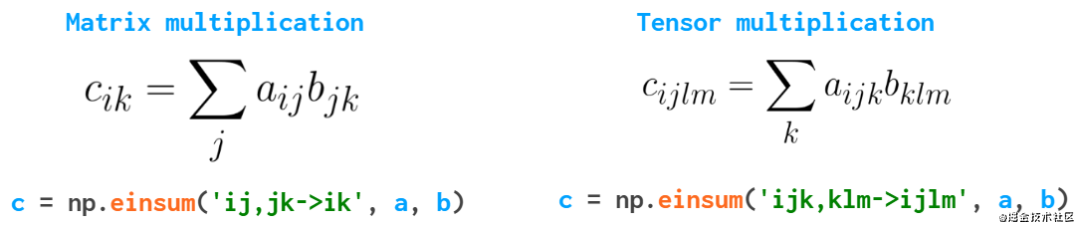## 评论